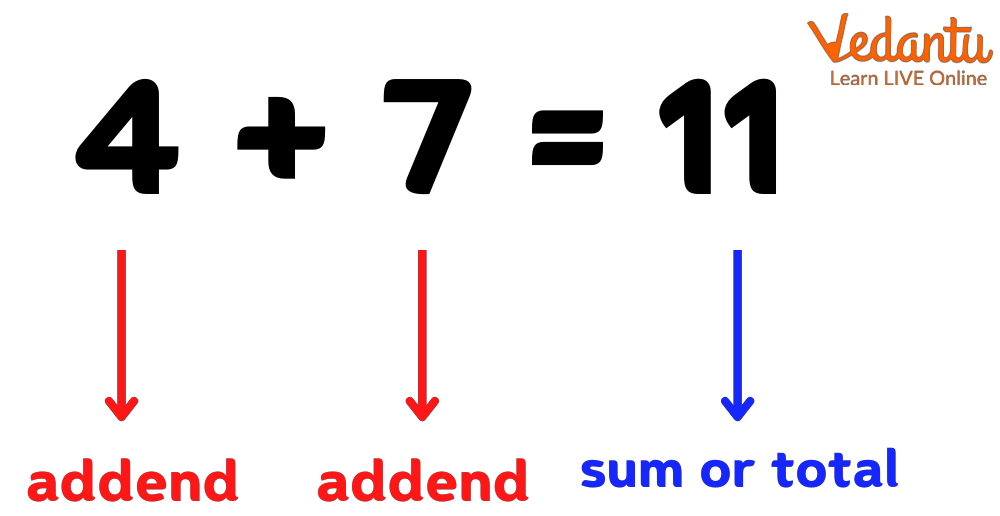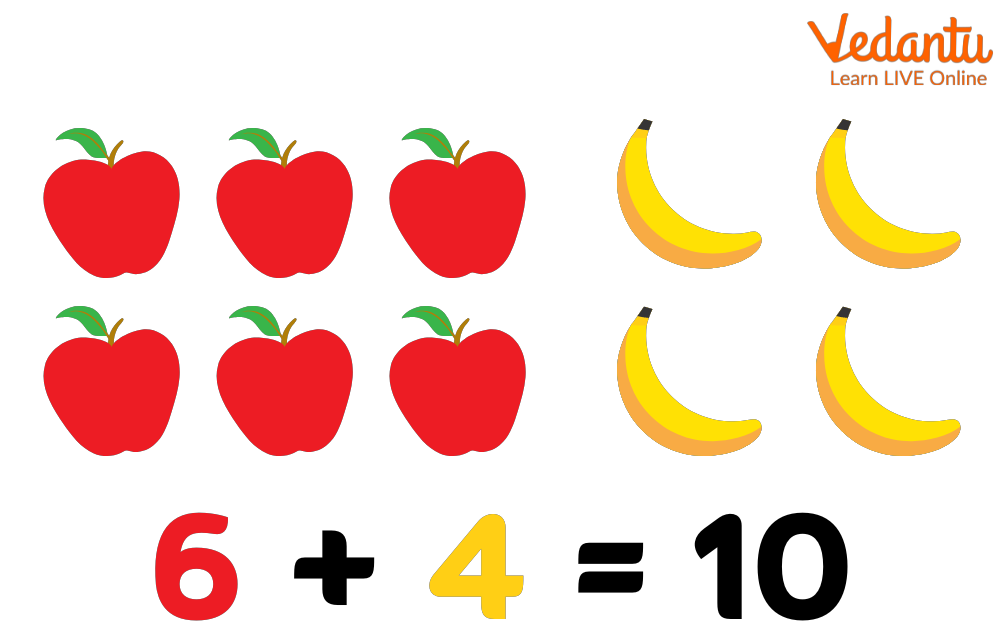Courses
Courses for Kids
Free study material
Free LIVE classes
More

# What is Sum?## Introduction to Sum

In mathematics, the phrase Sum refers to the result gained by adding numbers, objects, or things together. This word appears regularly in our daily lives. When we buy a particular quantity of products, such as fruits and vegetables, we ask the merchant to sum up the entire price we must pay. The whole amount is referred to as the sum in this case. You will discover the meaning of sum, how to find the sum of numbers in various scenarios, and examples in this article.

In mathematics, the sum is the result or response obtained by multiplying two or more numbers or words. As a result, the sum is a method of combining items. To put it another way, the sum is the process of adding two or more numbers to generate a new result or total.

They are taught to children in Math classes and might take the form of numerical sums or word problems.

A mathematical sum, often known as a mathematics sum, is the outcome of multiplying two or more integers. It's the sum of all the numbers put together. The total of 4 and 7 is 11, for example.## Some Example

A mathematical sum that employs visuals and symbols instead of numbers is another example. Each one can symbolize a different number; in this example, all of the fruits have the identical value of one. Sums with images are an excellent way to get young children interested in math in the EYFS. To get the total, young children can count each individual picture.Image: 6 Apple And 4 Bananas = 10

There are 6 apples and 4 bananas in these samples. Because we know that each piece of fruit has a value of one, we can simply count how many there are and add them up. 6+4=10 is the numerical sum.

## Summation Notation

When we add two integers together, we use the plus symbol (+). The sum is the label given to the outcome of addition. The total can be represented by the symbol ∑ (sigma). It's typically utilized when we need to add a long list or a series of numbers.

## Examples of Sum

Sums framed as word problems are useful for getting pupils to solve real-life mathematical issues. They can use money as an example in which they are asked to calculate the overall amount.

• Example 1- Peter has £42 and Bob has £50. What is the total amount of money they have if they pool their funds?

Ans: £92 is the correct answer.

• Example 2- There are 15 marbles in John's bag, 8 marbles in Mike's bag, and 17 marbles in Olivia's bag. What is the total value of their marbles?

Ans: 40 marbles

Last updated date: 16th Sep 2023
Total views: 135k
Views today: 3.35k

## FAQs on What is Sum?

1. What is the definition of sum in mathematics?

A sum is a result of adding two numbers together. When you put 1, 2, 3, and 4 together, you get the number 10, which is written. Addends, or summands, are the numbers being added together.

2. Is it correct to say that the total means to add?

The term total refers to the outcome of adding numbers, objects, or things in mathematics. For example, the sum of addends 14 and 6 equals 20.

3. What does the symbol for sum stand for?

The sum of a set of numbers is represented by the sigma (∑)symbol.